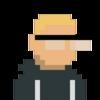## Welcome to the Treehouse Community

Want to collaborate on code errors? Have bugs you need feedback on? Looking for an extra set of eyes on your latest project? Get support with fellow developers, designers, and programmers of all backgrounds and skill levels here with the Treehouse Community!

### Looking to learn something new?

Treehouse offers a seven day free trial for new students. Get access to thousands of hours of content and join thousands of Treehouse students and alumni in the community today.# Altering an integer in a function

How do I alter the values in the point struct and then have it return that correctly? Thank you!

test.swift
```class Point {
var x: Int
var y: Int

init(x: Int, y: Int) {
self.x = x
self.y = y
}
}

enum Direction {
case left
case right
case up
case down
}

class Robot {
var location: Point

init() {
self.location = Point(x: 0, y: 0)
}

class Robot {
var location: Point

init() {
self.location = Point(x: 0, y: 0)
}

func move(_ direction: Direction) -> Point {
switch direction {
case Direction.left  : return Point(x: x - 1, y: y)
case Direction.right : return Point(x: x + 1, y: y)
case Direction.up    : return Point(x: x, y: y + 1)
case Direction.down  : return Point(x: x, y: y - 1)

}
}
}
```Hello mate. I was not sure what you are asking but I found some mistakes in your code. First, for some reason (or by mistake) you copy your Robot class inside of itself.

Second, in this function you wrote:

```    func move(_ direction: Direction) -> Point {
switch direction {
case Direction.left  : return Point(x: x - 1, y: y)
case Direction.right : return Point(x: x + 1, y: y)
case Direction.up    : return Point(x: x, y: y + 1)
case Direction.down  : return Point(x: x, y: y - 1)

}
}
```

Where you say: return Point(x: x - 1, y: y) you are passing a 'x' as an argument that doesn't exist. I know what you are trying to do, but you can't do like that.

Your third mistake was try to use Point to change the location of the Robot. You are supposed to use location to change the x and y of the robot since location is an instance of Point. You just need to update the x or y in the location instance, like this:

```class Robot {
var location: Point

init() {
self.location = Point(x: 0, y: 0)
}

func move(_ direction: Direction) {
switch direction {
case Direction.left:
return location.x -= 1
case Direction.right:
return location.x += 1
case Direction.up:
return location.y += 1
case Direction.down :
return location.y -= 1

}
}
}
```

I hope this helps.